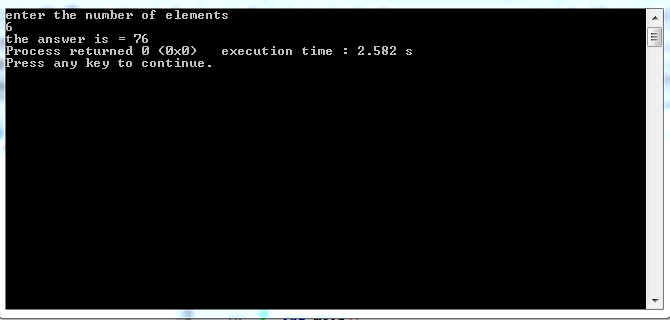×

Search anything:

# Number of ways to pair elements

#### Algorithms Dynamic Programming (DP)Get this book -> Problems on Array: For Interviews and Competitive Programming

Reading time: 25 minutes | Coding time: 5 minutes

In this article, we are going to discuss about element pairing problem where we need to find the number of ways a set of elements can be pair or kept separate. This can be solved using Dynamic Programming in linear time O(N).

What is the problem statement?

Consider we have 5 balls (1,2,3,4,5).

We are allowed to either pair them or keep them single. One ball can be paired only once in each sub-case. So our aim is to find the total number of ways of doing it.

For example:

let ball's are = [1,2,3]
cases:

``````[1,2,3]  none are paired.
[{1,2},3] 1 and 2 are paired.
[1,{2,3}] 2 and 3 are paired.
[{1,3},2] 1 and 3 are paired.
``````

so total no of ways are: 4

We cover this problem in the following sections:

• Intuition towards the solution
• Brute force approach
• Dynamic Programming

## Intuition towards the solution

If we consider the total number of ways of pairing or being single of 'n' distinct objects = C(N).

In our case for the 3rd ball there were two choices :

1. It can either be single, and so we repeat the process for the remaining 2 items.
so we actually do recursion.

2. It can pair with any one of the remaining 2 items .

so 1st case shows recursion for C(n-1) objects.

2nd case shows n-1* C(n-2) ways.

so keeping in mind the recursive factor we can write :

``````C(n) = C(n-1) + n-1* C(n-2)
``````

The base cases are:

``````C(1) = 1
C(0) = 1
``````

As if there is 1 or no element, there is only 1 combinations that is the input.

## Brute force approach

This brute force implementation uses the recursive mechanism to compute the relation that we have found.

``````C(n) = C(n-1) + n-1* C(n-2)
``````

Pseudocode:

``````int C(int i)
{
if(i == 0 || i == 1)
return 1;
return C(i-1) + (i-1) * C(i-2);
}
``````

Implementation:

``````#include<bits/stdc++.h>
using namespace std;

int brute_force(int i)
{
if(i==1)
return 1;
else if(i==2)
return 2;
return brute_force(i-1)+(i-1)*brute_force(i-2);
}

int main()
{
int no_ele;
cin>>no_ele;

cout<<brute_force(no_ele);
}
``````

This takes exponential time O(2^N) as the sub cases are computed multiple times. This can be optimized by using a Dynamic Programming approach.

## Dynamic Programming

While seeing the recursion and overlapping we actually get the vives of using dynamic programming.The idea that makes us think using dynamic programming is that we get are actually solving small parts of the problems and then summing the results for solving the biggers cases. This sub-problem soving ideas give us a will to use dynamic programming

So what we have to do is that:

1. create an array
2. we have to think of storing the value of number of possible ways of pairs/single of 'i' .
3. Now thsi where things get exciting, we are going to use the result of the previous values to calculate the nect values result, as we do in any typical DP solution, remembering the past, using it in future.
4. so its obvious that 2 will be the threshold base case.
5. And for values more than 2 we use the formula that we derived before.

Pseudocode:

``````int a[n + 1];
for (int i = 0; i <= n; i++)
{
if (i <= 2)
a[i] = i;
else
a[i] = a[i - 1] + (i - 1) * a[i - 2];
}
return a[n];
``````

The Dynamic programming approach:

``````#include <iostream>
using namespace std;
int dp-count(int n)
{
int a[n + 1];
for (int i = 0; i <= n; i++)
{
// this loop fills the array w.r.t the above dp formula
if (i <= 2)
a[i] = i;
else
a[i] = a[i - 1] + (i - 1) * a[i - 2];
}
return a[n];
}

int main()
{
cin>>n;
cout << dp_count(n);
return 0;
}
``````

output:step by step

``````at the start we have a empty array a[];
if we are evaluating for elements 1,2,3
so n=3;
so the loop runs from 0 to 3.

for i=0       //(i<=2);

a=0;

for i=1;     //(i<=2)

a=1;

for i=2;    //(i<=2)

a=2

for i=3;

a=a+2 * a[3-2]
a=2+2* 1;
a=4

so a which has a value of 4 is returned as the output.
``````

Complexity analysis

Time complexity : O(N)

Space complexity : O(N)

This was the dynamic programming approach, I hope it helped.

Happy coding :)#### Sourajeet Mohanty

Intern at OpenGenus | B. Tech student at College of Engineering and Technology, Bhubaneswar | Interested in Competitive programming and Blockchain

Number of ways to pair elements